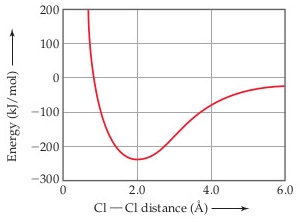# Problem: The following plot shows the potential energy of two Cl atoms as a function of the distance between them. We know that the Cl2 molecule exists. What is the approximate bond length for the Cl-Cl bond in Cl2 from this graph?

###### Problem Details

The following plot shows the potential energy of two Cl atoms as a function of the distance between them.We know that the Cl2 molecule exists. What is the approximate bond length for the Cl-Cl bond in Cl2 from this graph?